# How to Automatically Fill Increment Cells in Excel?

When dealing with Excel sheets, filling increment cells is a very regular task to complete. Cells that contain numerical values that are organized in a column according to a predetermined increment value are referred to as increment cells. This might be a series of sequential numbers such as 1, 2, 3, and so on, or it might be a series of numbers with fixed increments such as 12, 14, 16, 18 and so on.

In this tutorial, you are going to learn various solutions to automatically increment cells in excel.

## Using Autofill Function

Using the Autofill function, it is possible to automatically fill increment cells in Excel. The steps for doing so are as follows −

### Step 1

Choose a column, then in the first two cells of that column, insert the numerical values. The difference in the numerical values that are displayed in the two cells ought to be the increment value, and the cell that displays the lower value ought to be positioned above the cell that displays the larger value. See the following image.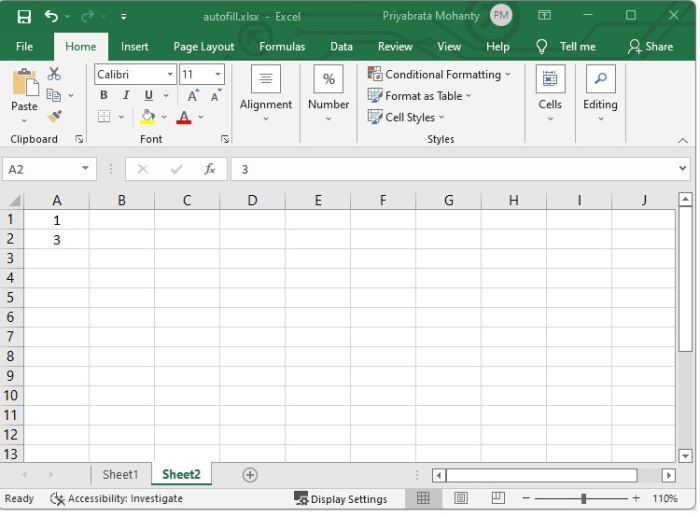In the above image, we have supplied "1" in A1 cell and "3" in A2 cell.

### Step 2

Now click outside the cell, and then pick those two cells once more. This will bring attention to the operation of Autofill.

To create an incremented list, locate the cell until which you need the selection to be pulled down and click on the dot that is located in the bottom-right corner of the selection. See the below given image.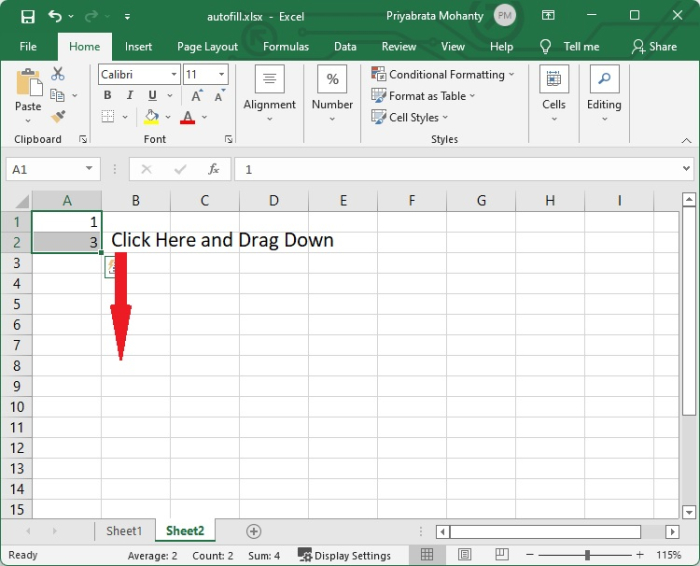In our example, we are incrementing the values by 2, that means the next incremented value should be 5, 7, 9, …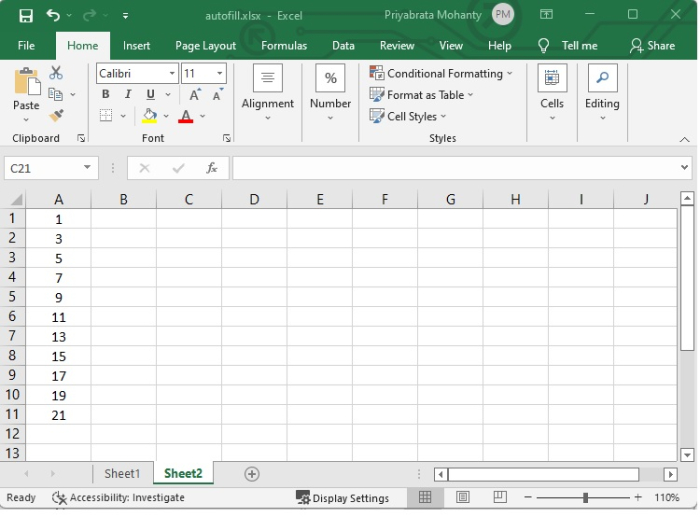## Using Formula

Even as you were working with the formula, you would have to make use of the Fill option. On the other hand, if you found yourself in this particular situation, you would not be adding things manually, but rather inputting the value of the increment. The formula for computing the increment value is as follows −

Starting cell of the list + increment value

Go through the following example to see how it is done.

### Step 1

Choose the cell in which you would like to enter your starting number. In our example, we have given 5 to A1 cell. See the below given image.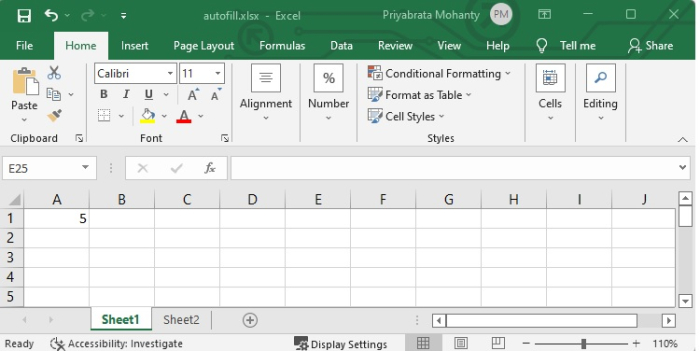### Step 2

Enter the following formula into the formula bar to get the desired output.

=(A1+5)

Here "A1" is the starting cell and "5" is the increment value. You can change them according to your requirement.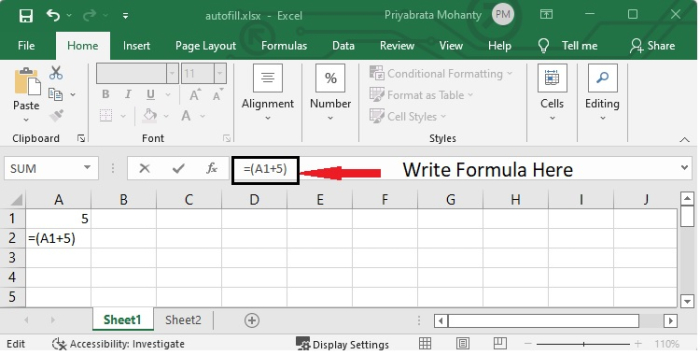After pressing Enter, you will see the incremented value in "A2" cell.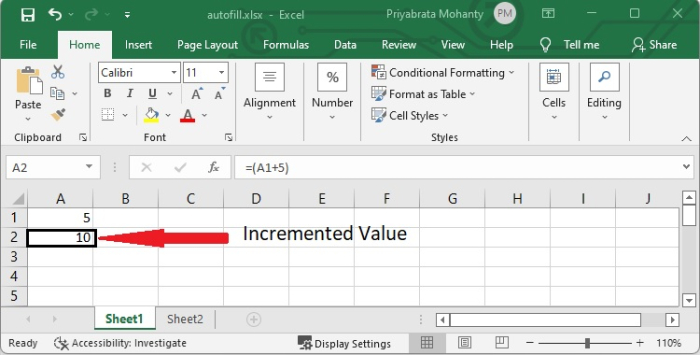### Step 3

Select that A2 cell and then drag this formula down to the cells that you want to be filled with numbers at a specified interval, and then drop it.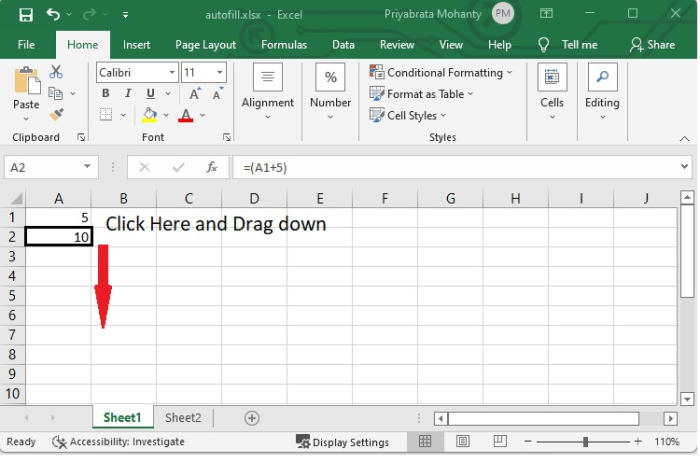Here is the final image of an incremented list of numbers.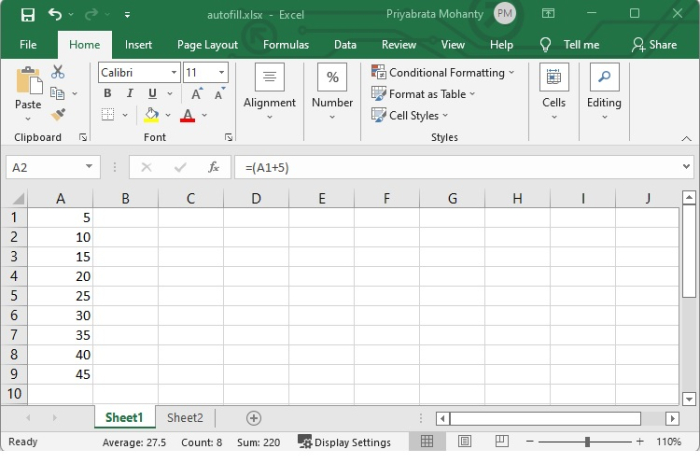## Conclusion

In this tutorial, you learnt two methods to fill incremented values in the cells automatically. However, you can use any of the methods according to your requirement.